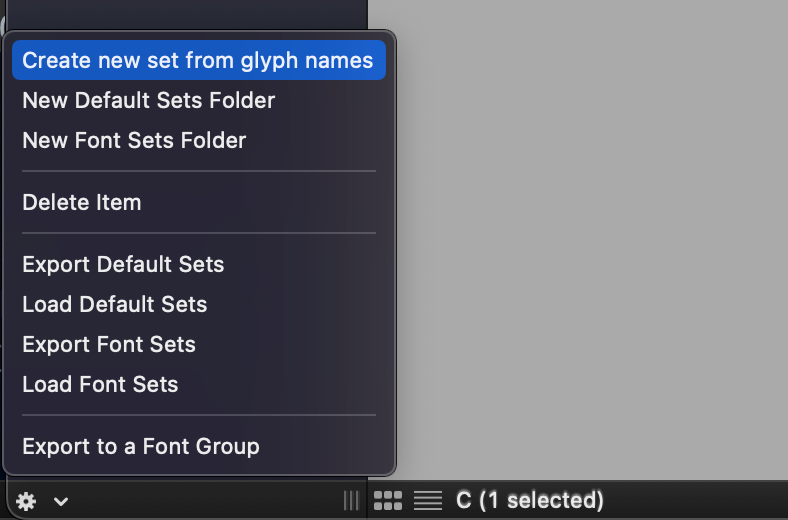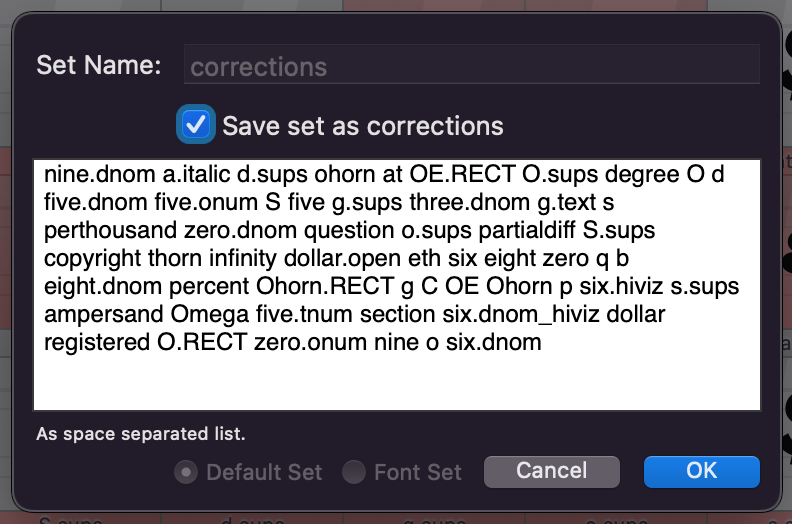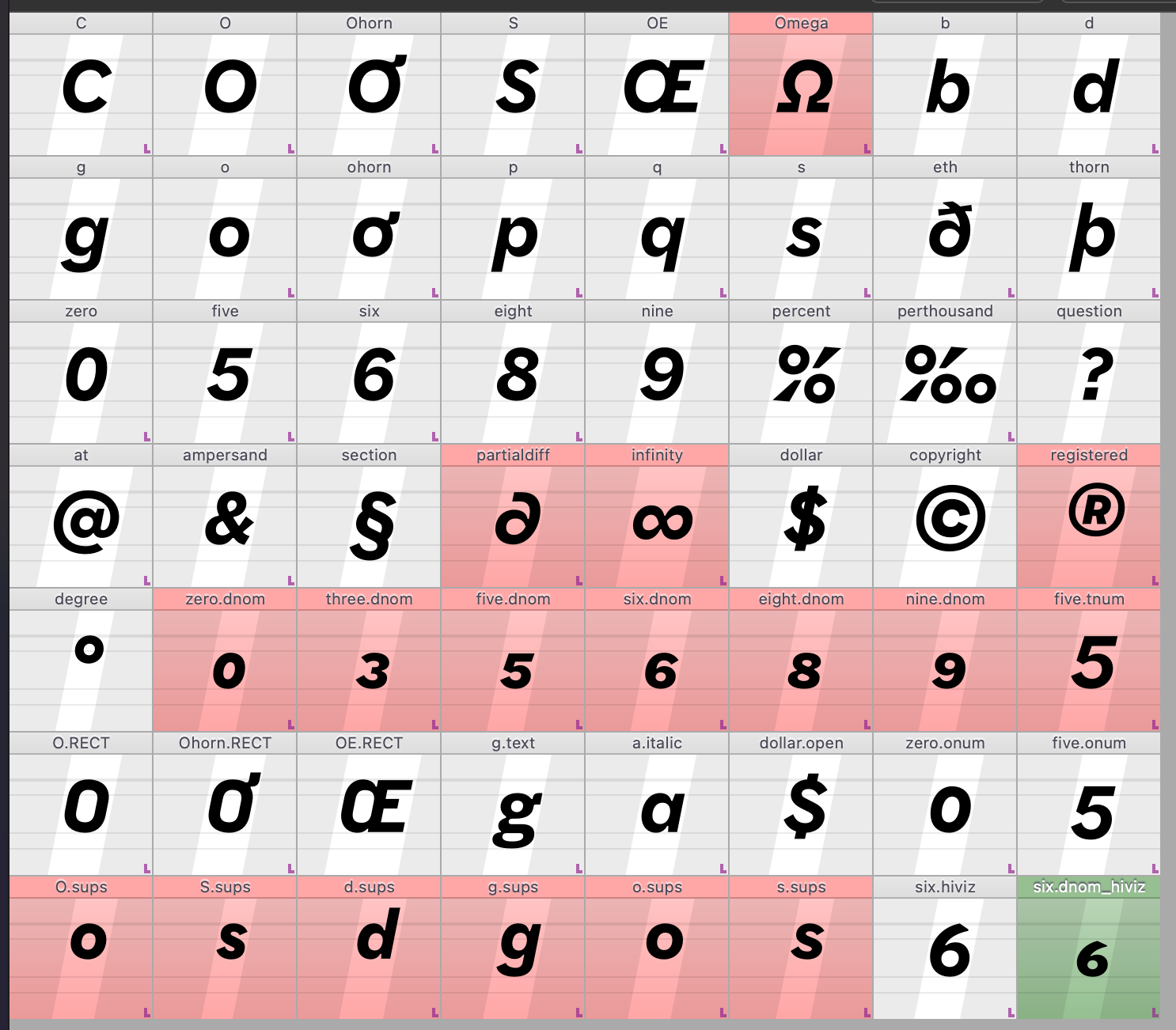# SOLVEDWhy are some start points becoming "2"? How might I fix it efficiently?

• If anyone (including my future self) comes along looking for a semi-manual solution, here’s a better one that what I listed above.

1. Open all the fonts you wish to check.
2. Run the following Python in the Scripting Window:
``````for f in AllFonts():
print(f.info.styleName)
glyphsToCheck = []
for g in f:
for contour in g:
pointCount = 0
for segment in contour:
for point in segment:
if pointCount == 0 and pointCount+1 != point.index:
if g.name not in glyphsToCheck:
glyphsToCheck.append(g.name)

pointCount += 1

print(" ".join(glyphsToCheck),"\n")

``````

This will print out a list to the console, like this:

``````Text Ultra Italic
eight registered

Text 200 Italic
thorn b a.italic question six d nine oe a p o copyright ringcomb g q

Text Hairline Italic
nine.dnom a.italic OE.RECT O.sups degree O d five.dnom five.onum S five three.dnom s zero.dnom question o.sups partialdiff S.sups copyright thorn infinity dollar.open eth six eight zero q b eight.dnom Ohorn.RECT g C OE p six.hiviz s.sups ampersand Omega five.tnum section six.dnom_hiviz dollar registered O.RECT zero.onum o six.dnom
``````

Then, you can "create a new set from glyphs names"Copy and paste in the glyph names, then label it with a name like "temp" or "corrections":This then makes it relatively fast to go through all the affect glyphs, using a shortcut to set the start point, then the shortcut to move to the next glyph in the font view `command+shift+)`.Once you finish, you can then delete the set.

I’m guessing there is a faster way to fix this, and it would probably be worth finding if you had many sources to fix... but for a small number of fonts, this is pretty quick.

• I am still interested if there is a programmatic way to set a specific oncurve start point, as asked above.

However, I may be facing this problem because FontMake added a new compatibility checker in v3.1.0, and this checker may be overly strict.

• Excellent; thanks for the helpful explanation, Frederik! That’s a really nice documentation page.

Do you know if there’s a fairly direct way to set the oncurve as the start point, in a Python script?

• the short answer: this is clarified in the upcoming release

https://robofont.com/documentation/reference/workspace/glyph-editor/contours/#point-representations

(this link will be gone in a few days, since the release is close)

long story:

The start point and the symbol to indicate the direction are not the same. In a UFO a contour can start with an off curve or an on curve and still represent the same visual contour:

``````# 3 times the same contour
off off curve line line
off curve line line off
curve line line off off
``````

The first on curve is not always the starting point. This was never a problem since fontMath does calculations nicely between different point structures. Other tools aren't so nice and choke.

RoboFont 4.2 has two symbols: one for the starting point and one for the direction (the direction is always highlighted on a on curve point)

hope this make sense!

• In the above GLIF code, both contour is messed up, but each contour should begin with one of the `type="curve" smooth="yes"` points.

``````			<point x="93" y="536" type="curve" smooth="yes"/>
<point x="93" y="189"/>
<point x="308.7858439201" y="-28"/>
``````

• The above is a simplified UFO with one glyph.

If you prefer just the .glif XML:

``````<?xml version="1.0" encoding="UTF-8"?>
<glyph name="o" format="2">
<unicode hex="006F"/>
<outline>
<contour>
<point x="308.7858439201" y="1102"/>
<point x="93" y="883"/>
<point x="93" y="536" type="curve" smooth="yes"/>
<point x="93" y="189"/>
<point x="308.7858439201" y="-28"/>
<point x="631" y="-28" type="curve" smooth="yes"/>
<point x="953.2141560799" y="-28"/>
<point x="1169" y="189"/>
<point x="1169" y="536" type="curve" smooth="yes"/>
<point x="1169" y="883"/>
<point x="953.2141560799" y="1102"/>
<point x="631" y="1102" type="curve" smooth="yes"/>
</contour>
<contour>
<point x="789" y="841"/>
<point x="868" y="731"/>
<point x="868" y="536" type="curve" smooth="yes"/>
<point x="868" y="341"/>
<point x="786" y="233"/>
<point x="631" y="233" type="curve" smooth="yes"/>
<point x="476" y="233"/>
<point x="395" y="341"/>
<point x="395" y="536" type="curve" smooth="yes"/>
<point x="395" y="731"/>
<point x="473" y="841"/>
<point x="631" y="841" type="curve" smooth="yes"/>
</contour>
</outline>
<anchor name="top" x="630" y="1072"/>
<anchor name="ogonek" x="810" y="0"/>
<anchor name="bottom" x="631" y="-28"/>
<guideline x="631" y="102" angle="90" color="0.999,0.001,0.001,0.39962" identifier="wGX5S9dabk"/>
</glyph>
``````

• I have a theory. Can you post a GLIF for me to test with?

• Hmm, it is possible this was a side-effect of a script I wrote to correct contour direction, so all exterior contours would have the same winding direction. Maybe the `c.reverse()` function will occasionally mess with the start point index?

``````for f in AllFonts():
for g in f:
try:
for c in g.contours:
if c.clockwise == True:
c.reverse()
except:
print(f"{g.name} couldn’t be wound counter-clockwise")
pass
``````

• I’ve at least been able to adapt my script slightly to point out problem glyphs, though I would still love to find an automatic fix.

``````g = CurrentGlyph()

for f in AllFonts():
print("\n", f.info.styleName)
for g in f:
for contour in g:
pointCount = 0
for segment in contour:
for point in segment:
if pointCount == 0 and pointCount+1 != point.index:
print(f"\t ⚠️ Glyph {g.name}, Contour {contour.index}")
contour.setStartSegment(contour[segment.index])

pointCount += 1
``````

This is currently yielding this result, showing that the problem is somewhat prevalent, though not horribly common:

``````
Display Bold
⚠️ Glyph g.sups, Contour 1
⚠️ Glyph o.sups, Contour 0
⚠️ Glyph o.sups, Contour 1
⚠️ Glyph partialdiff, Contour 0
⚠️ Glyph two, Contour 0
⚠️ Glyph o, Contour 0
⚠️ Glyph o, Contour 1

Text Ultra
⚠️ Glyph questiondown, Contour 0
⚠️ Glyph question, Contour 0
⚠️ Glyph three, Contour 0
⚠️ Glyph o.sups, Contour 0
⚠️ Glyph o.sups, Contour 1
⚠️ Glyph thorn, Contour 1
⚠️ Glyph six, Contour 0
⚠️ Glyph two, Contour 0
⚠️ Glyph section, Contour 1
⚠️ Glyph nine, Contour 0
⚠️ Glyph o, Contour 0
⚠️ Glyph o, Contour 1

Text Hairline
⚠️ Glyph questiondown, Contour 0
⚠️ Glyph g.sups, Contour 1
⚠️ Glyph question, Contour 0
⚠️ Glyph three, Contour 0
⚠️ Glyph o.sups, Contour 0
⚠️ Glyph o.sups, Contour 1
⚠️ Glyph thorn, Contour 1
⚠️ Glyph parenleft, Contour 0
⚠️ Glyph two, Contour 0
⚠️ Glyph section, Contour 1
⚠️ Glyph nine, Contour 0
⚠️ Glyph o, Contour 0
⚠️ Glyph o, Contour 1

Text Bold
⚠️ Glyph ohorn, Contour 0
⚠️ Glyph ohorn, Contour 1
⚠️ Glyph questiondown, Contour 0
⚠️ Glyph question, Contour 0
⚠️ Glyph three, Contour 0
⚠️ Glyph o.sups, Contour 0
⚠️ Glyph o.sups, Contour 1
⚠️ Glyph partialdiff, Contour 0
⚠️ Glyph thorn, Contour 1
⚠️ Glyph six, Contour 0
⚠️ Glyph two, Contour 0
⚠️ Glyph section, Contour 1
⚠️ Glyph nine, Contour 0
⚠️ Glyph o, Contour 0
⚠️ Glyph o, Contour 1

Display Ultra
⚠️ Glyph ohorn, Contour 0
⚠️ Glyph questiondown, Contour 0
⚠️ Glyph question, Contour 0
⚠️ Glyph o.sups, Contour 0
⚠️ Glyph o.sups, Contour 1
⚠️ Glyph partialdiff, Contour 0
⚠️ Glyph six, Contour 0
⚠️ Glyph two, Contour 0
⚠️ Glyph nine, Contour 0
⚠️ Glyph o, Contour 0
⚠️ Glyph o, Contour 1

Display Hairline
⚠️ Glyph questiondown, Contour 0
⚠️ Glyph oe, Contour 0
⚠️ Glyph question, Contour 0
⚠️ Glyph o.sups, Contour 0
⚠️ Glyph o.sups, Contour 1
⚠️ Glyph partialdiff, Contour 0
⚠️ Glyph thorn, Contour 1
⚠️ Glyph six, Contour 0
⚠️ Glyph nine, Contour 0
⚠️ Glyph o, Contour 0
⚠️ Glyph o, Contour 1
``````